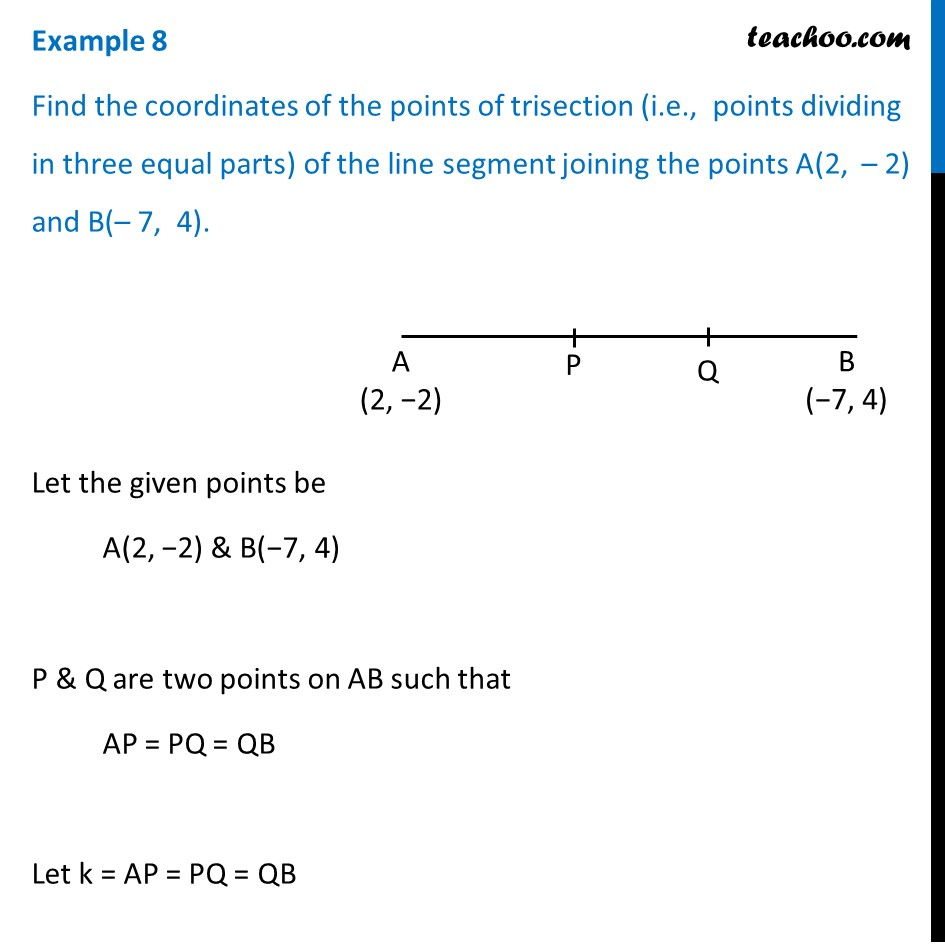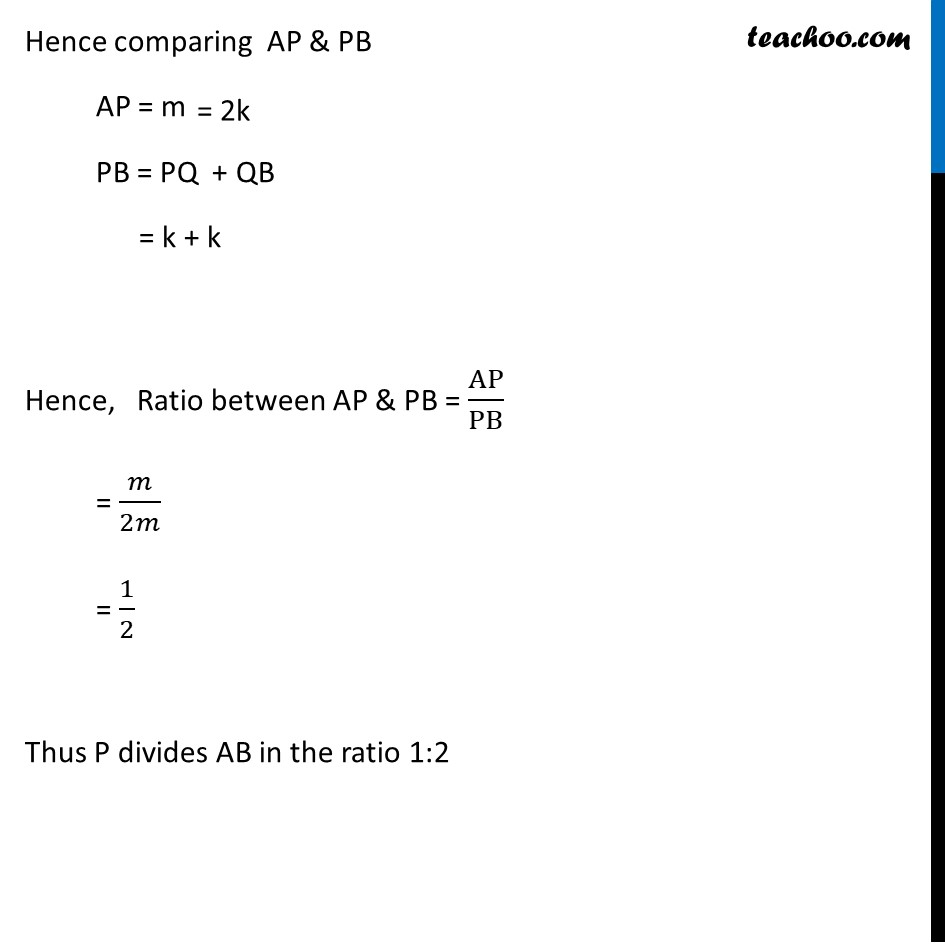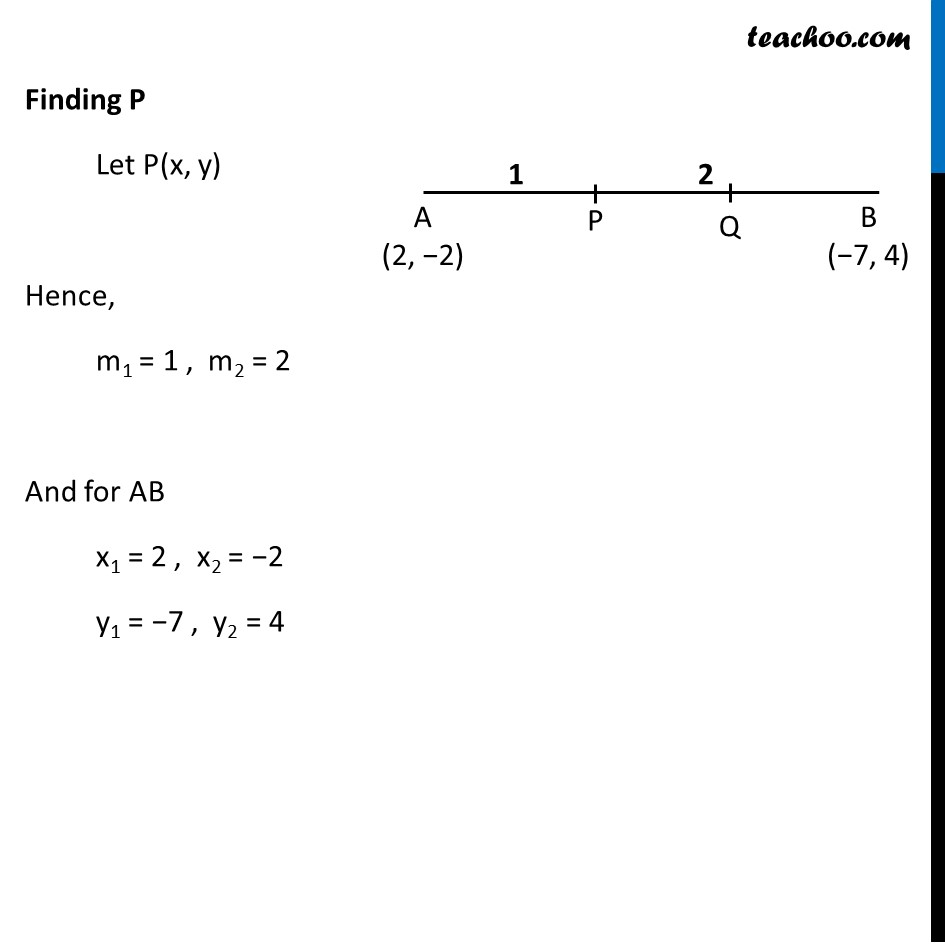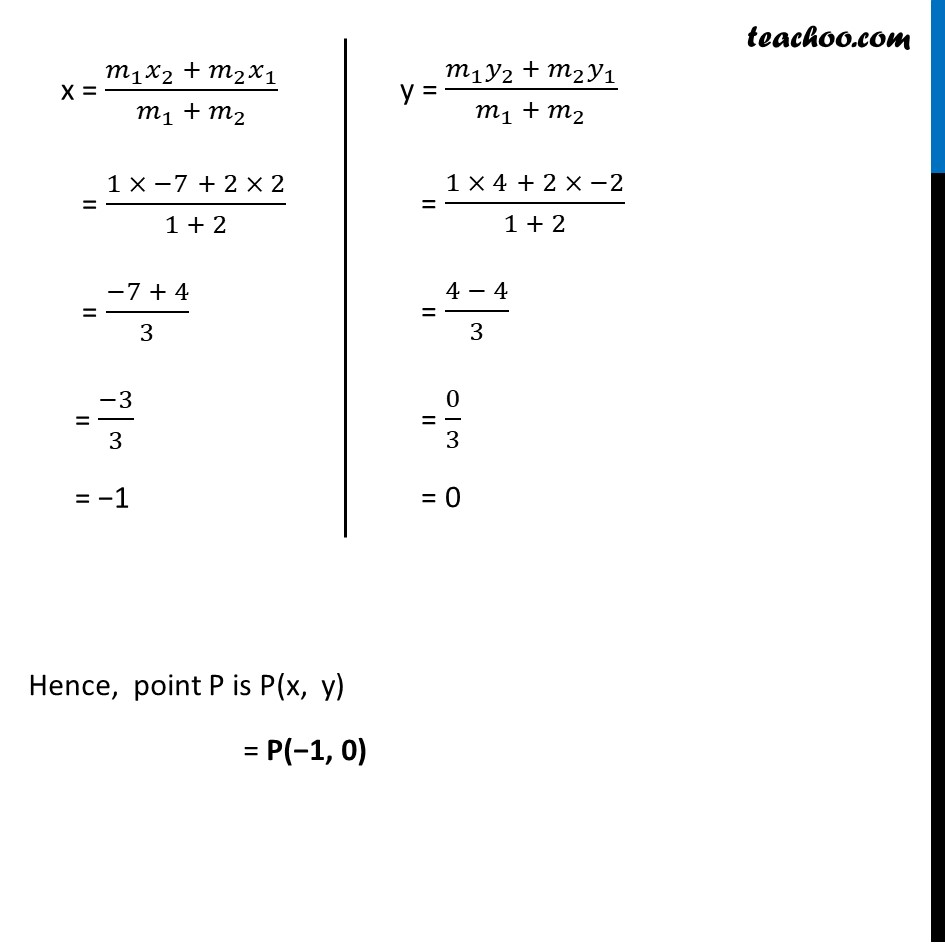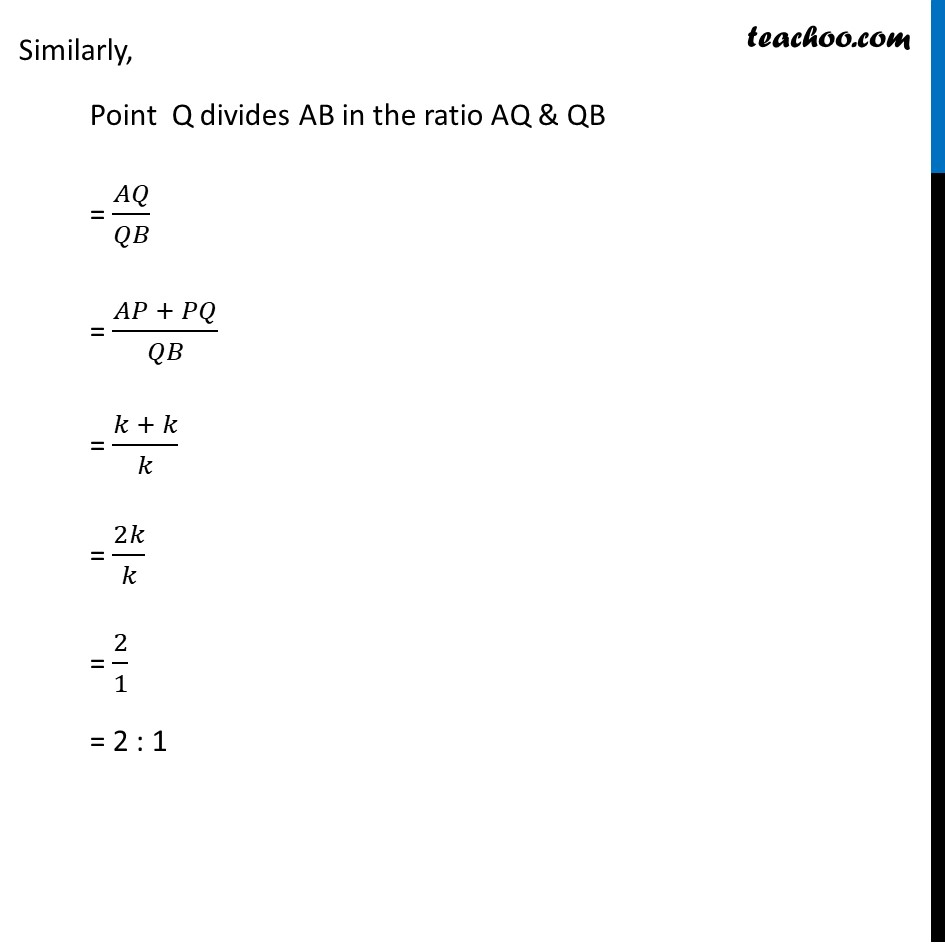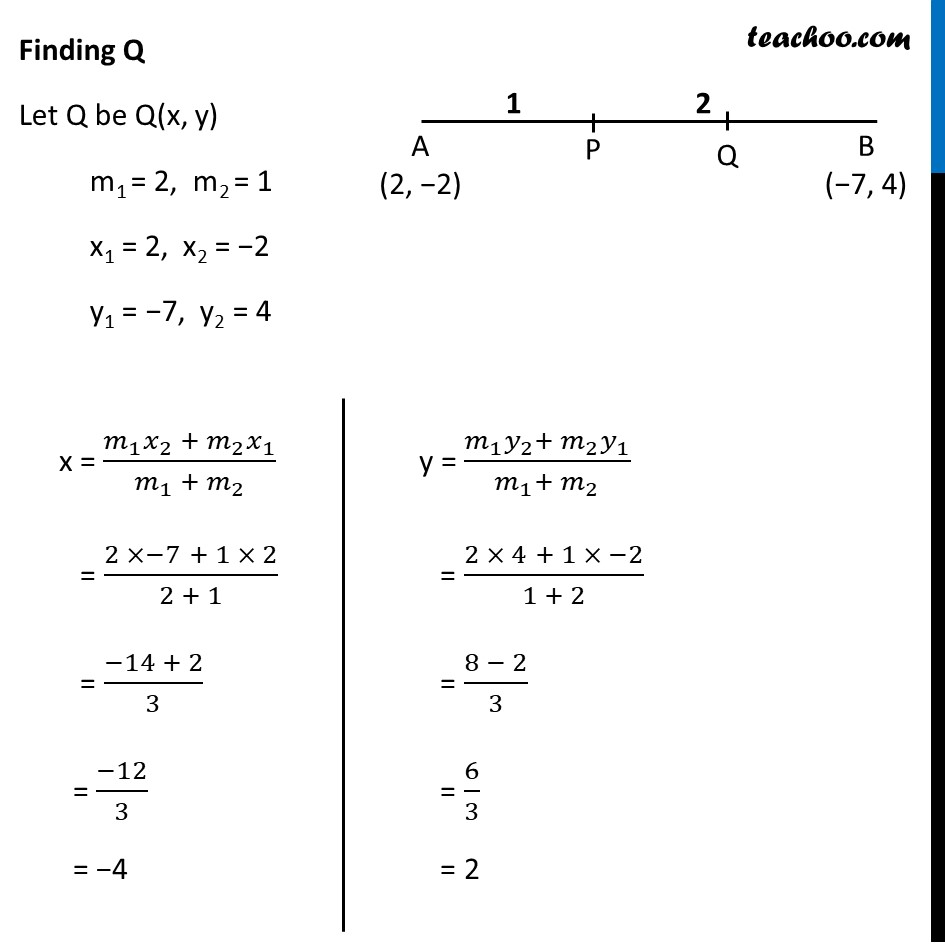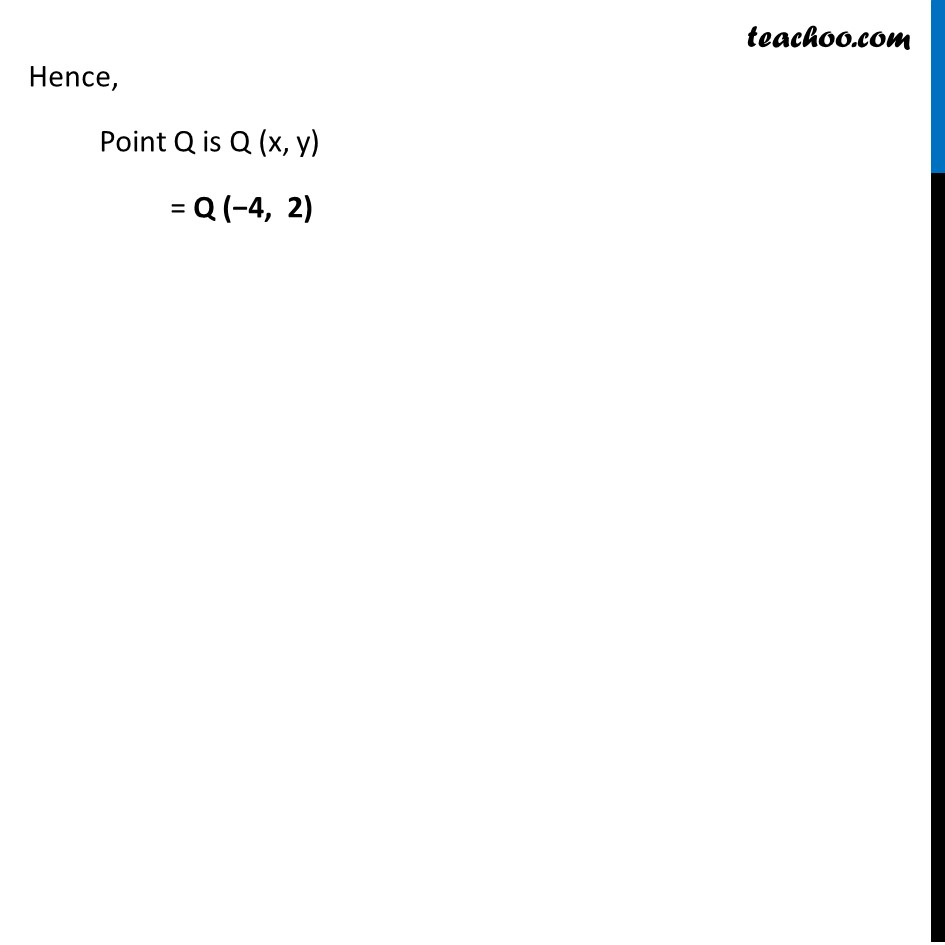1. Chapter 7 Class 10 Coordinate Geometry (Term 1)
2. Serial order wise
3. Examples

Transcript

Example 8 Find the coordinates of the points of trisection (i.e., points dividing in three equal parts) of the line segment joining the points A(2, – 2) and B(– 7, 4). Let the given points be A(2, −2) & B(−7, 4) P & Q are two points on AB such that AP = PQ = QB Let k = AP = PQ = QB Hence comparing AP & PB AP = m PB = PQ + QB = k + k Hence, Ratio between AP & PB = AP/PB = 𝑚/2𝑚 = 1/2 Thus P divides AB in the ratio 1:2 Finding P Let P(x, y) Hence, m1 = 1 , m2 = 2 And for AB x1 = 2 , x2 = −2 y1 = −7 , y2 = 4 x = (𝑚_1 𝑥_2 + 𝑚_2 𝑥_1)/(𝑚_1 + 𝑚_2 ) = (1 × −7 + 2 × 2)/(1 + 2) = (−7 + 4)/3 = (−3)/3 = −1 y = (𝑚_1 𝑦_2 + 𝑚_2 𝑦_1)/(𝑚_1 + 𝑚_2 ) = (1 × 4 + 2 × −2)/(1 + 2) = (4 − 4)/3 = 0/3 = 0 Hence, point P is P(x, y) = P(−1, 0) Similarly, Point Q divides AB in the ratio AQ & QB = 𝐴𝑄/𝑄𝐵 = (𝐴𝑃 + 𝑃𝑄)/𝑄𝐵 = (𝑘 + 𝑘)/𝑘 = 2𝑘/𝑘 = 2/1 = 2 : 1 Finding Q Let Q be Q(x, y) m1 = 2, m2 = 1 x1 = 2, x2 = −2 y1 = −7, y2 = 4 x = (𝑚_1 𝑥_2 + 𝑚_2 𝑥_1)/(𝑚_1 + 𝑚_2 ) = (2 ×−7 + 1 × 2)/(2 + 1) = (−14 + 2)/3 = (−12)/3 = −4 y = (𝑚_1 𝑦_2+ 𝑚_2 𝑦_1)/(𝑚_1+ 𝑚_2 ) = (2 × 4 + 1 × −2)/(1 + 2) = (8 − 2)/3 = 6/3 = 2 Hence, Point Q is Q (x, y) = Q (−4, 2)